Complex Ions

What is a complex metal ion?

A complex ion has a metal ion at its centre with a number of other molecules or ions surrounding it. These can be considered to be attached to the central ion by co-ordinate (dative covalent) bonds. (In some cases, the bonding is actually more complicated than that.)

As you know, a covalent bond is formed by two atoms sharing a pair of electrons. The atoms are held together because the electron pair is attracted by both of the nuclei. In the formation of a simple covalent bond, each atom supplies one electron to the bond - but that doesn't have to be the case.

A co-ordinate bond (also called a dative covalent bond) is a covalent bond (a shared pair of electrons) in which both electrons come from the same atom.

There is a bit of unique nomenclature to complex ions: The metal is known as the central metal ion. The anions or molecules attached to the metal are called ligands. The coordination number is the number of places on the metal ion where ligands are bound. The bond between the metal ion and the ligand, where the ligand supplies both electrons, is known as a coordinate covalent bond Simple ligands include water, ammonia and chloride ions.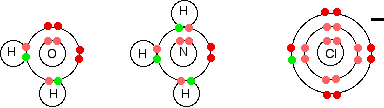What all these have got in common is active lone pairs of electrons in the outer energy level. These are used to form co-ordinate bonds with the metal ion.

All ligands are lone pair donors. In other words, all ligands function as Lewis bases.

Bonding in simple complex ions

Al(H2O)6 3+

We are going to look in detail at the bonding in the complex ion formed when water molecules attach themselves to an aluminium ion to give Al(H2O)63+.

Start by thinking about the structure of a naked aluminium ion before the water molecules bond to it.

Aluminium has the electronic structure

1s22s22p63s23px1

When it forms an Al3+ ion it loses the n=3 electrons:

1s22s22p6

That means that all the 3-level orbitals are now empty. The aluminium uses of six of these to accept lone pairs from six water molecules.It re-organises (hybridises) the 3s, the three 3p, and two of the 3d orbitals to produce six new orbitals all with the same energy.You might wonder why it chooses to use six orbitals rather than four or eight or whatever. Six is the maximum number of water molecules it is possible to fit around an aluminium ion (and most other metal ions). By making the maximum number of bonds, it releases most energy and so becomes most energetically stable.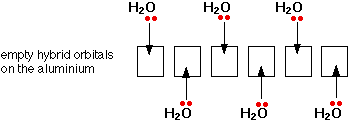Only one lone pair is shown on each water molecule. The other lone pair is pointing away from the aluminium and so isn't involved in the bonding. The resulting ion looks like this:Because of the movement of electrons towards the centre of the ion, the 3+ charge is no longer located entirely on the aluminium, but is now spread over the whole of the ion.Because the aluminium is forming 6 bonds, the co-ordination number of the aluminium is said to be 6. The co-ordination number of a complex ion counts the number of co-ordinate bonds being formed by the metal ion at its centre.

In a simple case like this, that obviously also counts the number of ligands - but that isn't necessarily so, as you will see later. Some ligands can form more than one co-ordinate bond with the metal ion.

Fe(H2O)6 3+

This example is chosen because it is very similar to the last one - except that it involves a transition metal.

Iron has the electronic structure

1s22s22p63s23p63d64s2

When it forms an Fe3+ ion it loses the 4s electrons and one of the 3d electrons

1s22s22p63s23p63d5

The orbital diagram looks like: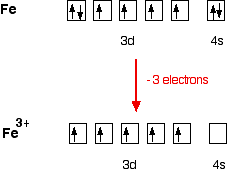Now, be careful! The single electrons in the 3d level are NOT involved in the bonding in any way. Instead, the ion uses 6 orbitals from the 4s, 4p and 4d levels to accept lone pairs from the water molecules.

Before they are used, the orbitals are re-organised (hybridised) to produce 6 orbitals of equal energy.

`  `Once the co-ordinate bonds have been formed, the ion looks exactly the same as the equivalent aluminium ion.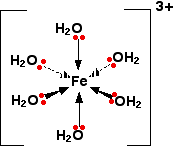Because the iron is forming 6 bonds, the co-ordination number of the iron is 6.

CuCl4 2-

This is a simple example of the formation of a complex ion with a negative charge.

Copper has the electronic structure

1s22s22p63s23p63d104s1

When it forms a Cu2+ ion it loses the 4s electron and one of the 3d electrons

1s22s22p63s23p63d9

To bond the four chloride ions as ligands, the empty 4s and 4p orbitals are used (in a hybridised form) to accept a lone pair of electrons from each chloride ion. Because chloride ions are bigger than water molecules, you can't fit 6 of them around the central ion - that's why you only use 4.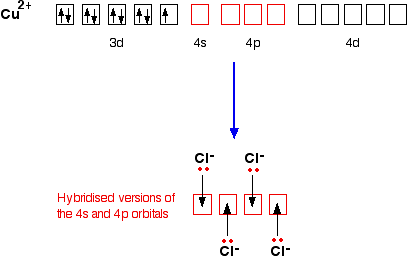Only one of the 4 lone pairs on each chloride ion is shown. The other three are pointing away from the copper ion, and aren't involved in the bonding.That gives you the complex ion:The ion carries 2 negative charges overall. That comes from a combination of the 2 positive charges on the copper ion and the 4 negative charges from the 4 chloride ions.

In this case, the co-ordination number of the copper is, of course, 4.

Coordination Numbers:

The total number of points of attachment to the central element is termed the coordination number and this can vary from 2 to as many as 16, but is usually 6. In simple terms, the coordination number of a complex is influenced by the relative sizes of the metal ion and the ligands and by electronic factors, such as charge which is dependent on the electronic configuration of the metal ion. These competing effects are described by the term ionic potential which is defined as the charge to radius ratio (q/r).

Based on this, it can be seen that the bigger the charge on the central ion, the more attraction there will be for negatively charged ligands, however at the same time, the bigger the charge the smaller the ion becomes which then limits the number of groups able to coordinate.

Typical Coordination Numbers:

Transition-metal complexes have been characterized with coordination numbers that range from 1 to 12, but the most common coordination numbers are 2, 4, and 6. Examples of complexes with these coordination numbers are given in the table below.

Examples of Common Coordination Numbers

 Metal Ion Ligand Complex Coordination Number Ag+ + 2 NH3 <=> Ag(NH3)2+ 2 Ag+ + 2 S2O32- <=> AgCl2- 2 Ag+ + 2 Cl- <=> Ag(S2O3)23- 2 Pb2+ + 2 OAc- <=> Pb(OAc)2 2 Cu+ + 2 NH3 <=> Cu(NH3)2+ 2 Cu2+ + 4 NH3 <=> Cu(NH3)42+ 4 Zn2+ + 4 CN- <=> Zn(CN)42- 4 Hg2+ + 4 I- <=> HgI42- 4 Co2+ + 4 SCN- <=> Co(SCN)42- 4 Fe2+ + 6 H2O <=> Fe(H2O)62+ 6 Fe3+ + 6 H2O <=> Fe(H2O)63+ 6 Fe2+ + 6 CN- <=> Fe(CN)64- 6 Co3+ + 6 NH3 <=> Co(NH3)63+ 6 Ni2+ + 6 NH3 <=> Ni(NH3)62+ 6

Note that the charge on the complex is always the sum of the charges on the ions or molecules that form the complex.

Cu2+ + 4 NH3 <=> Cu(NH3)42+

Pb2+ + 2 OAc- <=> Pb(OAc)2

Fe2+ + 6 CN- <=> Fe(CN)64-

Note also that the coordination number of a complex often increases as the charge on the metal ion becomes larger.

Cu+ + 2 NH3 <=> Cu(NH3)2+

Cu2+ + 4 NH3 <=> Cu(NH3)42+

Formation of Complex Ions:

The basic assumption behind the discussion of solubility equilibria is the idea that salts dissociate into their ions when they dissolve in water. Copper sulfate, for example, dissociates into the Cu2+ and SO42- ions in water.

 H2O CuSO4(s) <=> Cu2+(aq) + SO42-(aq)

If we add 2 M NH3 to this solution, the first thing we notice is the formation of a light-blue, almost bluish-white, precipitate. This can be explained by combining what we know about acid-base and solubility equilibria. Ammonia acts as a base toward water to form a mixture of the ammonium and hydroxide ions

 NH3(aq) + H2O(l) <=> NH4+(aq) + OH-(aq) Kb = 1.8 x 10-5

The OH- ions formed in this reaction combine with Cu2+ ions in the solution to form a Cu(OH)2 precipitate.

 Cu2+(aq) + 2 OH-(aq) <=> Cu(OH)2(s) Ksp = 2.2 x 10-20

In theory, the OH- ion concentration should increase when more base is added to the solution. As a result, more Cu(OH)2 should precipitate from the solution. At first, this is exactly what happens. In the presence of excess ammonia, however, the Cu(OH)2 precipitate dissolves, and the solution turns deep-blue. This raises an important question: "Why does the Cu(OH)2 precipitate dissolve in excess ammonia?"

The first step toward answering this question involves writing the electron configuration of copper metal and its Cu2+ ion.

 Cu = [Ar] 4s1 3d10 Cu2+ = [Ar] 3d9

It is sometimes useful to think about the electron configuration of the Cu2+ ion in terms of the entire set of valence-shell orbitals. In addition to the nine electrons in the 3d subshell, this ion has an empty 4s orbital and a set of three empty 4p orbitals.

Cu2+ = [Ar] 4so 3d9 4po

The Cu2+ ion can therefore pick up pairs of nonbonding electrons from four NH3 molecules to form covalent Cu-N bonds,

Cu2+(aq) + 4 NH3(aq) <=> Cu(NH3)42+(aq)

as shown in the figure below.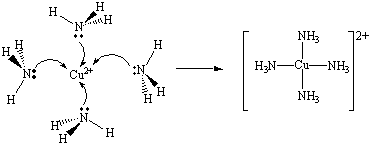The formation of a complex ion is a stepwise process and each step has its own characteristic equilibrium constant.

For the formation of Ag(NH3)2+, the reactions are: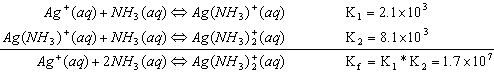Notice: when two equations are added together, the equilibrium constants multiply.

Cations that form complex ions with excess NH3 are:

1.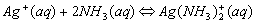2.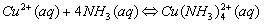3.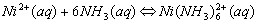4.Cations that form complex ions with excess OH- are:

1.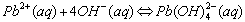2.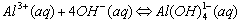3.The formation of a complex ions is suspected when:

1. The addition of a reagent causes precipitate formation followed by dissolving of the precipitate on addition of excess reagent.

OR

1. The formation of a colored species in solution is observed.

 Formation constants of complexes Complexes have an equilibrium constant for their formation like any other chemical reaction. For example, for the complex Cd(OH)4-2, the formation reaction is Cd+2(aq) + 4OH-(aq) -> Cd(OH)4-2(aq) and therefore the equilibrium constant expression is K = [Cd(OH)4-2] / [Cd+2][OH-]4 The equilibrium constant is often called the formation constant for the reaction. Example: What is the formation constant expression for the complex Co(NH3)5NO2+2? Solution: Just write out the formation reaction and then write the equilibrium constant expression for that reaction. The formation reaction is Co+3(aq) + 5NH3(aq) + NO2-(aq) -> Co(NH3)5NO2+2 Therefore, the equilibrium constant expression (formation constant) is K = [Co(NH3)5NO2+2] / ([Co+3][NH3]5[NO2-])